AsTher Thermodynamic Database and Applications

AsTher Process Calculator for MS Excel

5.4. Enthalpy-Concentration, Liquid-Gas-Concentration-Diagrams of Ethanol-Water Mixtures

This example shows the sheets of the MS Excel file to plot state diagrams using the empirical equations of activity coefficients in the application AsTher Process Calculator.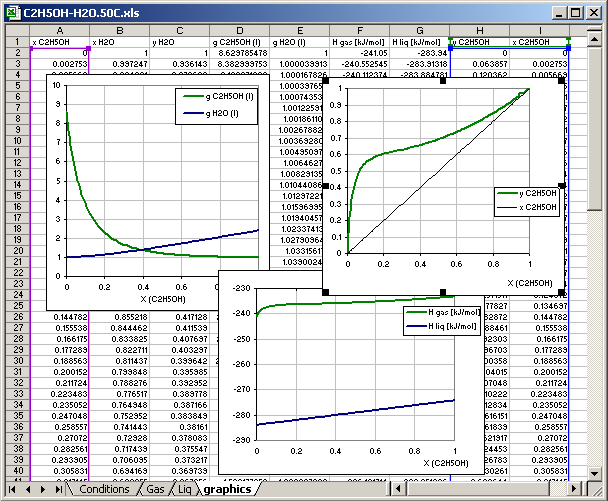The C2H5OH-H2O.50C.xls contains 4 sheets: conditions, Gas, Liq and graphics.
The data in the sheets are written in following

5.4.1. Conditions Sheets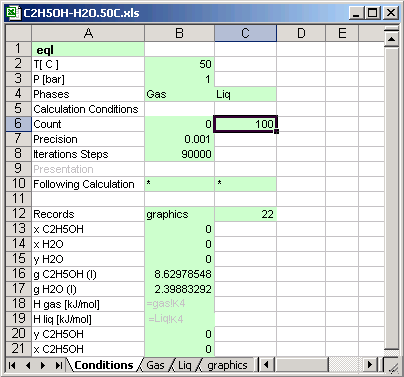Cell [B2] Temperature of the thermodynamic system
Cell [B3] Pressure of the thermodynamic system
Cell [B6] Calculation is made 100 time, the input values changed in depend of the calculation count for different concentrations of the substances.

Cell [B10] * : means if the calculation count is less than 100 [C6], then the next calculations system is defined in the same file.
Cell [C10] * : means if the calculation count is less than 100 [C6], then the next calculations system is defined in the same condition sheet.

Cell [B12] graphics: Calculations result is written in the sheet named graphics, the sheet must exist in the same file (or in the same MS Excel book)

Cells [B13] to [B21]: the cells contain links to another cell in different sheet, which data are recorded in the sheet  graphics

5.4.2. Sheet for gas phase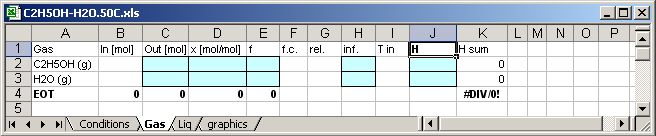In cell [J1] written H, so that the application writes enthalpies of the substances in the cells [J2] and [J3].
Enthalpy of the C2H5OH in the mixture [K2]=C2*J2
Enthalpy of the H2O in the mixture [K3]=C3*J3
Molar Enthapy of the mixture [K4]=(K2+K3)/C4
Mass of the mixture in mol [C4]=C3+C4

The calculation results are written in the blue coloured cells,

5.4.3. Sheet for liquid phase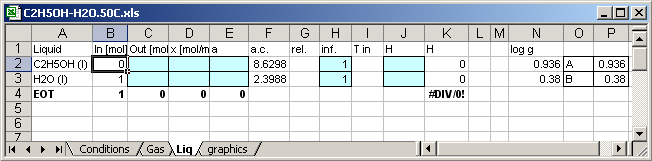The cell [B2]=Conditions!B6*0.01 the input values are varied in depend of the calculation count.
The cell [B2]=1-B2  the input values are varied in depend of the calculation count.

Enthalpy of the C2H5OH in the mixture [K2]=C2*J2
Enthalpy of the H2O in the mixture [K3]=C3*J3
Molar Enthapy of the mixture [K4]=(K2+K3)/C4
Mass of the mixture in mol [C4]=C3+C4

In cell [J1] written H, so that the application writes enthalpies of the substances in the cells [J2] and [J3].

The activity coefficients of the binary mixtures given by Van Laar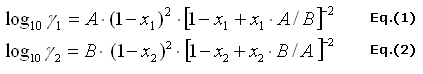There are Eq.(1) the activity coefficients of ethanol and Eq.(2) the activity coefficient of water in the binary mixture.

The constants A and B are given by C.A. Jones, A.P. Schoenborn, Ind. Chem. Eng. 40 (1940) 1273-1276
for 50 C
A is in Cell [P2]=0.936
B is in Cell [P3]=0.38

Eq.(1) and Eq.(2) written in Cells [N2] and [N3]

[N2]=P2*POWER(1-D2;2)*POWER((1-D2+D2*P2/P3);-2)    Eq. (1)
[N3]=P3*POWER(1-D3;2)*POWER((1-D3+D3*P3/P2);-2)    Eq. (2)

The cells [F2] and [F3] contain the activity coefficients:
[F2]=POWER(10;N2)
[F3]=POWER(10;N3)

5.4.4. Calculations
When Process Calculator for MS Excel runs, and button Calculation is pressed, the calculations are carried out 100 time for different input values. The Result of the calculation is written in the sheet graphics.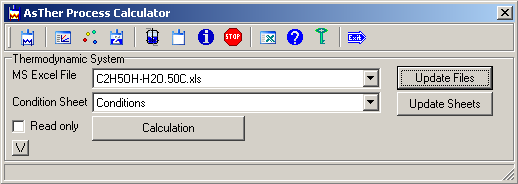5.4.5. Sheet for Graphics

The sheet graphics contains no any data before the calculations.
The data in th colums A.. I are written by application corresponding the parameters in the conditions sheet.